# Area of Circle

Go back to  'Area'

Consider  a circle of radius $$r$$ :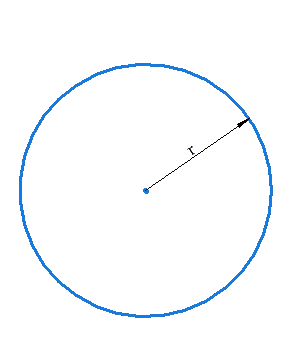We know that

• The perimeter (length of the circumference) of this circle will be $$2\pi r$$ units.

• The area of this circle will be $$\pi {r^2}$$ square units.

## What is pi ?

Before jumping into the technicalities, let’s familiarize ourselves with the diameter and the perimeter or circumference of a circle. To visualize the circumference, think of the crust of a Pizza, well, that’s essentially what it is! The length of the outer edge of any circle.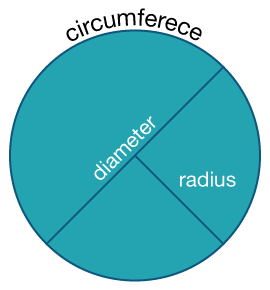Here’s where things get really interesting. What if you were told that if you divide the circumference of ANY circle with its diameter, you will ALWAYS end up with the SAME number, 3.1415…

The use of ellipses is deliberate! This particular ratio is always the same regardless of the circle in question! Yes, it is mind-boggling, which is why this number occupies such a crucial role in all of the mathematics related to the circle. This ratio is called Pi.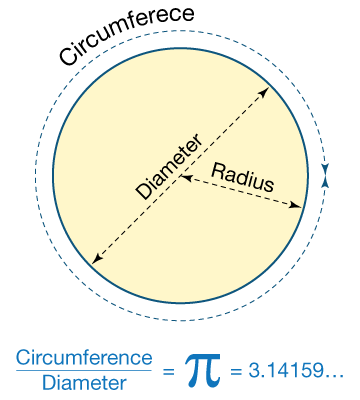Regarding the number, pi is a non-repeating, non-terminating entity which is best approximated to the fraction $${22 \over 7}$$. The decimal representation up to 10 places is 3.1428571429

## How is it important?

### Area of a Circle

What if you need to find out how much space the lawn covers to that you can find out the amount to be paid to a gardener who charges by the area he has mown? Or if you want to find out the space covered by the circular pizza; you will need to find out its area. The area of a circle again is a function of π and its radius, but the radius is considered in two dimensions.

$${\text{Area of a circle = }}$$$${πr^2}$$

Since the Diameter D of a circle is twice the radius, therefore we can also replace the radius r by $${D \over 2}$$ in the above formula and get

$${\text{Area of a circle = }}$$$${πD^2 \over 4}$$

When we ask the question, “How much space does the pizza enclose?” What is implied may be visualized using the image below. The space enclosed is represented by the shaded (blue) area.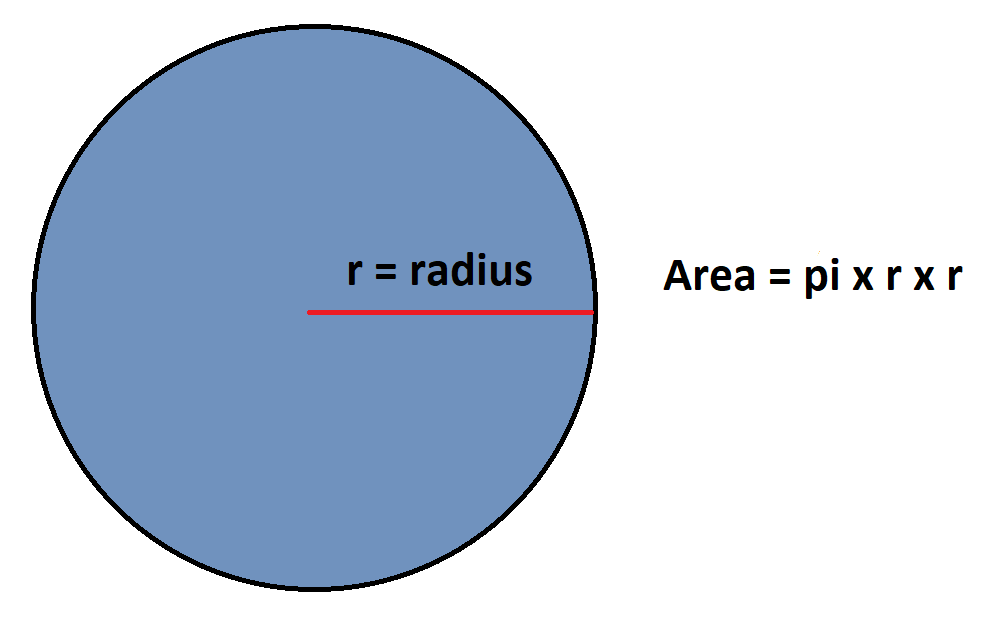What about the area of a semi-circle?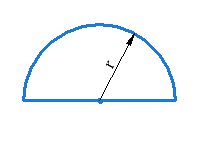The area of this semi-circle will be $$\frac{1}{2}\pi {r^2}$$, while the perimeter will be

\begin{align}&\frac{1}{2}{\rm{ of circumference + diameter}}\\&{\rm{ = }}\pi {{r + 2r}}\\&{\rm{ = }}\left( {\pi + 2} \right)r\end{align}

Now ,consider a circular sector of radius $$r$$ and angle $${\theta ^ \circ }$$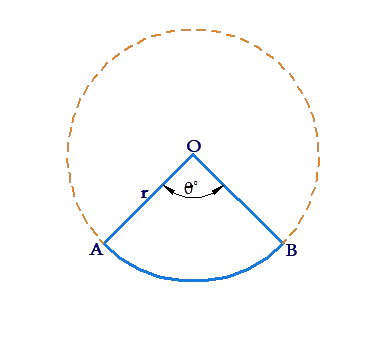Let us calculate the perimeter and area of this sector. Note that a circle is a perfectly symmetrical figure about its center. The complete circumference subtends an angle of $${360^ \circ }$$  at the center. If an arc subtends an angle $${\theta ^ \circ }$$ at the center, its length  will be in the same proportion to the circumference as $${\theta ^ \circ }:{360^ \circ }$$. A similar remark applies for the area of the circular sector. Thus:

•     Perimeter

\begin{align}& P = OA + OB +\widehat{AB}\\ & \quad= r + r + \frac{{{\theta ^\circ }}}{{{{360}^\circ}}} \times 2\pi r\\ &\quad= 2r\left( {1 + \frac{{\pi \times {\theta ^\circ }}}{{{{360}^\circ}}}} \right)\end{align}

•      Area

\begin{align}A = \frac{{{\theta ^ \circ }}}{{{{360}^ \circ }}} \times \pi {r^2}\end{align}

Note that if we denote the arc length of $$\widehat{AB}$$  by $$l$$, then

$l = \frac{{2\pi r \times {\theta ^ \circ }}}{{{{360}^\circ }}}$

In terms of $$l$$, the area of the circular sector can be written as: $A = \frac{1}{2} \times l \times r$

Make sure you understand this .

Next we consider how to evaluate the areas of circular segments. In the  following figure, chord $$AB$$ divides the circle into two segments, a minor segment (containing the minor arc $$\widehat{AB}$$ ), and a major arc (containing the major arc$$\widehat{AB}$$  ):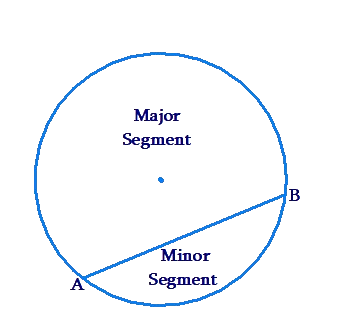The question is: given a chord of a circle, how can we evaluate  the areas of the two segments created by that chord ?

In the following figure, chord $$AB$$ subtends an angle of $${\theta ^ \circ }$$ at the circle’s center. Let us evaluate the area of the  minor segment created by $$AB$$: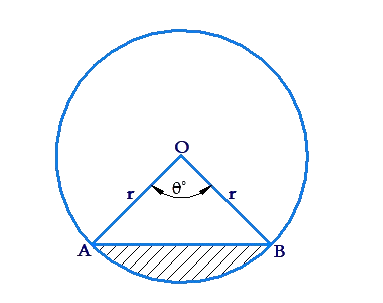We can write the required area as $area\left( {{\rm{sector OAB}}} \right) - area\left( {\Delta OAB} \right)$

The area of (minor) sector $$OAB$$ will be

\begin{align}\frac{{{\theta ^ \circ }}}{{{{360}^ \circ }}} \times \pi {r^2}\end{align}

Let us calculate the area of $$\Delta OAB$$. In the figure below, we have redrawn $$\Delta OAB$$. OC is perpendicular to  $$AB$$. Note that $$C$$ must be mid-point of $$AB$$: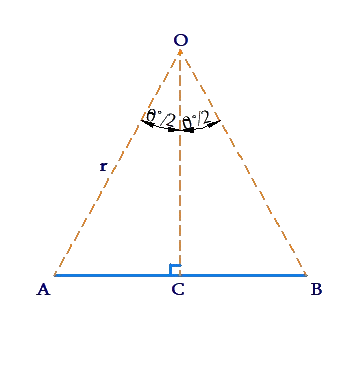We have:

\begin{align}&AC = r\sin {\left( {\frac{\theta }{2}} \right)^\circ}\\&OC = r\cos {\left( {\frac{\theta }{2}} \right)^\circ }\end{align}

Thus,

\begin{align}& Area\left( {\Delta OAB} \right) = \frac{1}{2} \times AB \times OC\\ &\qquad\qquad\qquad \;= AC \times OC\\&\qquad\qquad\qquad \; = {r^2}\sin {\left( {\frac{\theta }{2}} \right)^\circ}\cos {\left( {\frac{\theta }{2}} \right)^\circ }\\ &\qquad\qquad\qquad \;= \frac{1}{2}{r^2}\left\{ {2\sin {{\left( {\frac{\theta }{2}} \right)}^\circ }\cos {{\left( {\frac{\theta }{2}} \right)}^\circ}} \right\}\\&\qquad\qquad\qquad \;\ = \frac{1}{2}{r^2}\sin {\theta ^\circ} \end{align}

We have made use of the fact that

$$\sin 2A = 2\sin A\cos A$$

We can now conclude that the areas of the minor segment $$AB$$ is

${\Delta _{\min }} = \frac{{{\theta ^ \circ }}}{{{{360}^ \circ }}} \times \pi {r^2} - \frac{1}{2}{r^2}\sin {\theta ^ \circ }$

The area of the major segment $$AB$$ can now simply be calculated as

${\Delta _{maj}} = \pi {r^2} - {\Delta _{\min }}$

Example 1: A wire, when bent into a square form,  encloses an area of 100 cm2. It is now straightened out and re-bent into a semi-circular form as shown below. What is the area of this semi-circle?

Solution: The side of the square is 10 cm, and hence  the length of the circle is the  perimeter of this square, or 40cm.

Now,  if the radius of the semi-circle is $$r$$, we have :

\begin{align}&\pi r + 2r = 40\;cm\\& \Rightarrow r = \frac{{40}}{{\pi + 2}} \approx 7.8\;cm\end{align}

The  area enclosed by this semi-circle is $A = \frac{1}{2}\pi {r^2} \approx 95.5\;c{m^2}$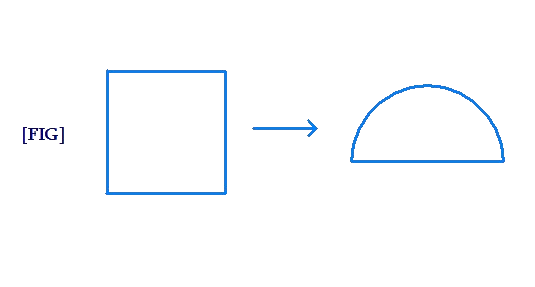Example 2: A chord of a circle is 21 cm long, and makes  a right angle at the  center. Find the area of  the minor segment of the circle  created  by this chord.

Solution: consider the following figure: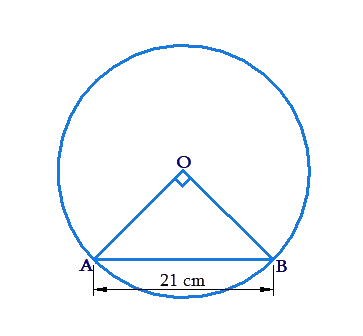If the radius of the circle is $$r$$, we have in $$\Delta OAB$$ :

\begin{align}&O{A^2} + O{B^2} = {r^2} + {r^2} = {21^2}\\& \Rightarrow r = \frac{{21}}{{\sqrt 2 }}{\rm{} \;cm}\end{align}

Now,

\begin{align}&area(minor\; sector\;OAB)\\&{\rm{ = }}\frac{{{\theta ^\circ}}}{{{{360}^\circ }}} \times \pi {r^2}\\ &\approx \left( {\frac{{{{90}^\circ }}}{{{{360}^\circ }}} \times \frac{{22}}{7} \times \frac{{441}}{2}} \right)c{m^2}\\& = 173.25\;c{m^2}\\&area\left( {\Delta OAB} \right)\\ &= \frac{1}{2} \times OA \times OB\\& = \frac{1}{2}{r^2}\\ &= \left( {\frac{1}{2} \times \frac{{441}}{2}} \right)c{m^2}\\& = 110.25\;c{m^2}\end{align}

Taking the difference of these two areas ,the area of the minor segment made by $$AB$$ is 63 cm2.

Example 3: Consider  the following figure: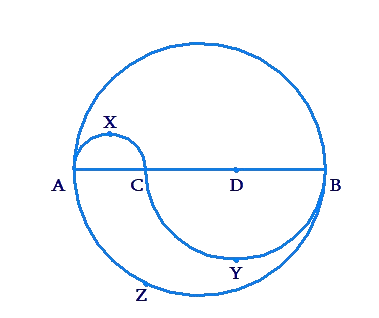$$AB$$ is a diameter of the circle, trisected by $$C$$ and $$D$$. On AC as diameter, semi-circle  $$AXC$$  is constructed . On $$BC$$ as diameter, semi-circle $$BYC$$  is constructed find the area of the shaded region, if $$AB = 3\;l$$ cm.

Solution: In the  half of the bigger circle above $$AB$$, the area of the shaded  region is the area of semi-circle $$AXC$$ , or

\begin{align}&\frac{1}{2} \times \pi \times {\left( {\frac{{AC}}{2}} \right)^2} = \frac{1}{2} \times \pi \times {\left( {\frac{l}{2}} \right)^2}\\ &\qquad\qquad\qquad\qquad= \left( {\frac{{\pi {l^2}}}{8}} \right)c{m^2}\end{align}

Below $$AB$$, the area of the shaded region is

\begin{align}& \text{area}(\text{semi - circle}\ AZB)\text{- area}(\text{semi - circle}\ CYB) \\ & \text{=}\left\{ \frac{1}{2}\times \pi \text{ }\!\!~\!\!\text{ }\times {{\left( \frac{AB}{2} \right)}^{2}} \right\}-\left\{ \frac{1}{2}\times \pi \text{ }\!\!~\!\!\text{ }\times {{\left( \frac{CB}{2} \right)}^{2}} \right\} \\ & =\left\{ \frac{1}{2}\times \pi \text{ }\!\!~\!\!\text{ }\times {{\left( \frac{3l}{2} \right)}^{2}} \right\}-\left\{ \frac{1}{2}\times \pi \text{ }\!\!~\!\!\text{ }\times {{\left( \frac{2l}{2} \right)}^{2}} \right\} \\ & =\frac{9\pi {{l}^{2}}}{8}-\frac{\pi {{l}^{2}}}{2} =\left( \frac{5\pi {{l}^{2}}}{8} \right)c{{m}^{2}} \\ \end{align}

The total area of  the shaded region is

$\frac{{\pi {l^2}}}{8} + \frac{{5\pi {l^2}}}{8} = \left( {\frac{{3\pi {l^2}}}{4}} \right)c{m^2}$

Example 4:  Consider the following figure: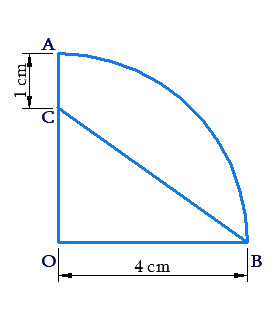$$OAB$$ is a quadrant  of a circle.  Find the area of the shaded region.

Solution: The required area is

\begin{align}& area(quadrant\ OAB)-\text{area}(\Delta OCB) \\& \text{=}\left( \frac{1}{4}\times \pi \text{ }\!\!~\!\!\text{ }\times {{4}^{2}} \right)-\left( \frac{1}{2}\times 4\times 3 \right)(how?) \\ & =4\pi \text{ }\!\!~\!\!\text{ }-6 \approx \text{ }\!\!~\!\!\text{ }6.56c{{m}^{2}} \end{align}

Example  5: $$\Delta ABC$$ is a right-angled at $$B$$, with $$AB$$ = 48 cm and $$BC$$=14 cm. A semi-circle is drawn with $$AC$$ as diameter. With $$BC$$ as radius ,a quadrant of a circle is drawn: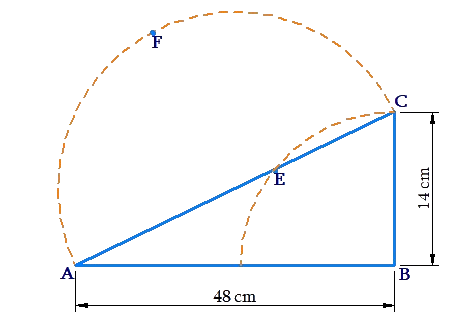Find the area of the shaded region .

Solution: By the Pythagoras Theorem in $$\Delta ABC$$,  we note that

\begin{align}&AC = \sqrt {A{B^2} + B{C^2}} = \sqrt {{{48}^2} + {{14}^2}} \\ &\qquad\qquad\qquad\qquad\quad= 50\;cm\end{align}

The required area is

\begin{align}& area\left( \Delta ABC \right)+area\left( \text{semi-circle }\,AFC \right)-area\left( \text{quadrant}\ DEC \right) \\ & =\left( \frac{1}{2}\times 48\times 14 \right)+\left( \frac{1}{2}\times \pi \text{ }\!\!~\!\!\text{ }\times {{25}^{2}} \right)-\left( \frac{1}{4}\times \pi \text{ }\!\!~\!\!\text{ }\times {{14}^{2}} \right)\approx 1163.4\;c{{m}^{2}} \end{align}

Example 6: $$\Delta ABC$$ is a right -angled at $$A$$, with $$AB$$ =6cm, and $$BC$$ =10cm. Find the area enclosed within $$\Delta ABC$$ external  to its incircle.

Solution: Consider the following figure, which shows the incircle of $$\Delta ABC$$ with center $$O$$ :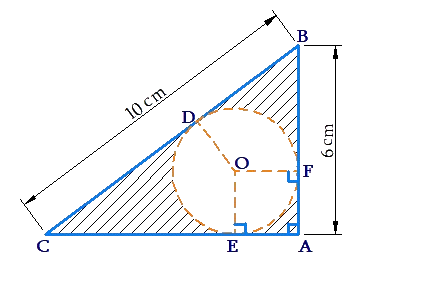Our problem is to find out the radius of the incircle, which will enable us to calculate the area of the shaded region.

Note that

$AC = \sqrt {B{C^2} - A{B^2}} = 8\;cm$

Suppose that the radius of the incircle is $$r$$ cm, We make the following observatiosns:

•       $$AE=AF=r \;\text{cm}$$
•        $$BF=(6-r)\,cm,\text{ }\!\!~\!\!\text{ }\,\,\,\text{CE}\,\text{=}\,(\text{8}-r)\,\text{cm}$$
•        \left\{ \begin{align}& BD=BF=(6-r)\,cm \\ & CD=CE=(8-r)\,cm \end{align} \right\}\left( why? \right)

We also have  :

\begin{align}&BD + CD = BC = 10\; cm\\& \Rightarrow \left( {6 - r} \right) + \left( {8 - r} \right) = 10\\& \Rightarrow r = 2 \;cm\end{align}

Thus, the area of the shaded region is

\begin{align}&area\left( {\Delta ABC} \right) - area\left( {incircle} \right)\\ &= \left( {\frac{1}{2} \times 8 \times 6} \right) - \left( {\pi \times {2^2}} \right) \approx 11.4c{m^2}\end{align}

Example 7: Consider the following  figure: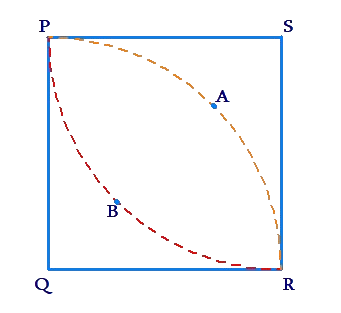$$PQRS$$  is a square. $$QPAR$$ is a quadrant of a circle with $$QP$$ as radius. $$SRBP$$ is also a quadrant of a circle with $$SR$$ as  radius. Find the ratio of the area of the shaded region to the area of the square.

Solution: Let each  side of the square be $$r$$ units long. The area of the square is $${{r}^{2}}$$  square units. Now, let us calculate the area of the shaded region $$PBRQ$$.

This area is equal to

\begin{align}&area(\text{square}\ PQRS)-\text{area}\left( \text{quadrant}\ SRBP \right) \\&\text{ }\!\!~\!\!\text{ }={{r}^{2}}-\frac{1}{4}\times \pi \text{ }\!\!~\!\!\text{ }\times {{r}^{2}} \\& \text{ }\!\!~\!\!\text{ }=\left( 1-\frac{\pi }{4} \right){{r}^{2}} \end{align}

Thus, the total area of the scheduled region is  twice of this area, or

$A = 2\left( {1 - \frac{\pi }{4}} \right){r^2}$

The required ratio is

$\frac{A}{{{r^2}}} = 2\left( {1 - \frac{\pi }{4}} \right) \approx 0.43$

Example 8: A regular hexagon is inscribed in a circle. Let $$X$$ be the area internal to the circle but external to the hexagon. Let $$H$$ be the area of the hexagon. Find $$\frac{X}{H}$$ .

Solution: consider the following figure: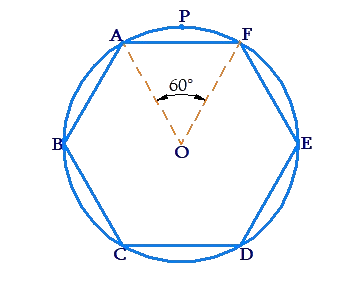Note that $$X$$ is the total area of the shaded region. Let the radius of the circle be $$r$$ units. we have :

\begin{align}& area\left( \Delta OAF \right)=\frac{1}{2}\times OA\times OF\times \sin {{60}^{^{{}^\circ }}}\text{ }~\text{ } \\ & \qquad \qquad \qquad =\frac{1}{2}{{r}^{2}}\times \frac{\sqrt{3}}{2} \\ & \qquad \qquad \qquad =\frac{\sqrt{3}}{4}{{r}^{2}}sq.units \\ & area(sector\ OAF)=\frac{{{60}^{^{{}^\circ }}}}{{{360}^{^{{}^\circ }}}}\times \pi {{r}^{2}} \\ & \qquad \qquad \qquad \qquad =\frac{\pi }{6}{{r}^{2}}sq.units \\ & \Rightarrow area(\text{minor}\ \text{segement}\,AF) \\ &\text{=area}(\text{sector}-OAF)\text{area}(\Delta OAF) \\ & \ \ \text{=}\left( \frac{\pi }{6}-\frac{\sqrt{3}}{4} \right){{r}^{2}}sq.units \\ \end{align}

Thus,

\begin{align} & \frac{X}{H}=\frac{6\times area(\text{minor}\ \text{segment}\,AF)}{6\times \ area(\Delta OAF)} \\ & \quad =\frac{\left( \frac{\pi }{6}-\frac{\sqrt{3}}{4} \right){{r}^{2}}}{\frac{\pi }{6}{{r}^{2}}} \\ & \quad =1-\frac{3\sqrt{3}}{2\pi } \\ \end{align}

The approximate value of this ratio is 0.17, which makes sense because the area of the shaded region is much less as compared to the area of hexagon.

Perimeter and Area